﻿ Use of FEM in Identification of Stress in Place of Concentration Factor

### Use of FEM in Identification of Stress in Place of Concentration Factor

Ingrid Delyová, Darina Hroncová, Peter Sivák

American Journal of Mechanical Engineering

## Use of FEM in Identification of Stress in Place of Concentration Factor

Ingrid Delyová1,, Darina Hroncová2, Peter Sivák1

1Department of Applied Mechanics and Mechanical Engineering, Faculty of Mechanical Engineering Technical University of Košice, Slovakia

2Department of Mechatronics, Faculty of Mechanical Engineering Technical University of Košice, Slovakia

### Abstract

Stress concentrators are sudden changes of shape of mechanic part, which evoke changes of force flow, so they influence tensions, which load this part. The same goes for the static or the cyclic loading. The notches which are on the surfaces of various components increase the stress. Such kind of stress creates instability and weakening of the component. However, it is possible to reduce that influence by adding such notches which are spaced apart in some distance.

• Ingrid Delyová, Darina Hroncová, Peter Sivák. Use of FEM in Identification of Stress in Place of Concentration Factor. American Journal of Mechanical Engineering. Vol. 4, No. 7, 2016, pp 454-459. http://pubs.sciepub.com/ajme/4/7/41
• Delyová, Ingrid, Darina Hroncová, and Peter Sivák. "Use of FEM in Identification of Stress in Place of Concentration Factor." American Journal of Mechanical Engineering 4.7 (2016): 454-459.
• Delyová, I. , Hroncová, D. , & Sivák, P. (2016). Use of FEM in Identification of Stress in Place of Concentration Factor. American Journal of Mechanical Engineering, 4(7), 454-459.
• Delyová, Ingrid, Darina Hroncová, and Peter Sivák. "Use of FEM in Identification of Stress in Place of Concentration Factor." American Journal of Mechanical Engineering 4, no. 7 (2016): 454-459.

 Import into BibTeX Import into EndNote Import into RefMan Import into RefWorks

1234
Prev Next

### 1. Introduction

The stress concentration factors cause the sudden change of component shape which induce the change of the force flow around the component . The uniaxial state of stress is changing to triaxial state of stress (rotating rod) or to biaxial (thin flat rod). The notches can be divided into three main groups:

• The Construction notches, eg.: the grooves, the recesses, the threads, etc.

• The Technological notches (Macrodefects of material).

• The Metallurgical notches, eg.: significant distortions of the internal structure, the dirt, etc.

Just the construction notches are desirable. From the construction and the operation point of view is the concentration undesirable because it has big influence on the life cycle of the whole structure .

### 2. The State of Stress and the Deformation around the Notches

The first works which were concentrated on the determination of the state of stress and the deformation around the notches were provided by Neuber. According the theoretical analysis the level of stress around the notches depends on the radius of curvature around the root of notch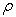, the side of angels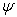, and also it depends on the depth of the notch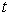. Neuber has developed the stress concentration factor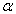to characterize the concentration of stress around the notches . The value of that factoris proportional to the maximum level of stress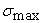and inversely proportional to the nominal (mean) stress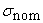, Figure 1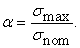(1)

The shape factor takes into account the increase of the stress because of the geometrical effect of the components and also it reflect the detailed change of the force flow component.Download asVeiw figureFigures index
Figure 1. Stress in components with notches
2.1. The Influence of Notches to Fatigue Strength

The notches which are on the surfaces of various components increase the stress. Such kind of stress creates instability and weakening of the component. However, it is possible to reduce that influence by adding such notches which are spaced apart in some distance. It is caused by the stress concentration factor which is for above situation lower than one notch case. Simply, the group of notches are acting like there is one notch with a larger radius of root curvature . To determinemoderating effect of these notches characterized as the depth of the notch which we can count from the following formula: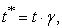(2)

Where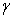is mitigating factor andis the depth of then notch.depends of ratio of separation notches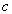and the depth of the notchwhich is shown on Figure 2.Download asVeiw figureFigures index
Figure 2. Dependence of ratio of separation notches and the depth of the notch

The shape factor of notchfor the component with more than one notch can be calculated from the known formulas. To determinefor the flat bar with a rectangular cross-section which is under the tensile stress we can use following formula, Figure 3 .Download asVeiw figureFigures index
Figure 3. Multiple opposite semicircular notches in finite-width plate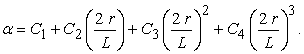(3)

Constants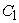to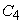are known from: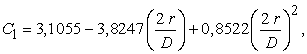(4)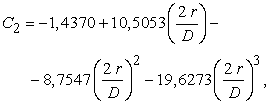(5)(6)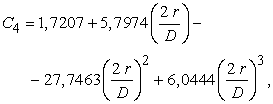(7)

where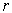is radius of notch,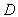is width of sample , L is distance between the notches.

2.2. Determination of Fatigue Strength for the Alternating Symmetrical Loading

The fatigue strength for each type of loading can be determined from the favorable conditions which can be modified by such factors, which can reduce the fatigue strength. Such factors are the surface treatment, notch, and also sensitivity of notch.

The fatigue strength can be determined from the below formulas for the alternating symmetrical tensile loading or bending loading and also of the torque loading: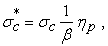(8)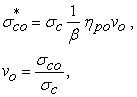(9)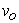is factor of size by bending,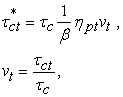(10)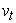is factor of size by torsion.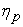is quality factor of surface, which we can determine from the graph Figure 4. The value of that factor for the bend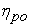is almost the same like the value of. In case of torque moment, we need to modify that factor according the following formula: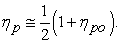(11)Download asVeiw figureFigures index
Figure 4. Quality factor of surface
2.3. Tensile Stress

We have used the same method of calculating the loading force F for the case with the three sided notches as we used for one sided notch case of static and alternating symmetrical tensile loading. However, there is one difference when it comes about the factor determination process. We have calculated that factor from the formula (3). The component was modeled at various mutual distances of notches L. The distances were chosen as follow 40 mm, 50 mm, 55 mm and 65 mm. According the formula (3), there will be different value ofonce the distance L will be changed.

The results can be found in the following table Table 1. You can also find the results of the static loading model on the Figure 5, Figure 6Download asVeiw figureFigures index
Figure 5. Field of reduced stress with 40 mm of notches range by the tensileDownload asVeiw figureFigures index
Figure 6 Field of reduced stress with 50 mm of notches range by the tensileDownload asVeiw figureFigures index
Figure 7. Field of reduced stress with 55 mm of notches range by the alternating symmetrical tensileDownload asVeiw figureFigures index
Figure 8. Field of reduced stress with 65 mm of notches range by the alternating symmetrical tensile

For the alternating symmetrical tensile loading, the results are shown on Figure 7, Figure 8.

2.4. Bending Stress

In case of component with the three sided notches was the value of loading force F also counted in the same way as we used for one notch case. The valuewas determined in the same way for both types also. According the change of mutual distance of adjacent notches we were able to observe the change ofparameter.

The following Table 1 provides our results. The results of modeling for the static loading are shown at Figure 9 and Figure 10.

The Figure 11 and Figure 12 provides the results of modeling the alternating symmetrical loading.Download asVeiw figureFigures index
Figure 9. Field of reduced stress with 40 mm of notches rangeby the bendingDownload asVeiw figureFigures index
Figure 11. Field of reduced stress with 55 mm of notches range by the alternating symmetrical bendingDownload asVeiw figureFigures index
Figure 12. Field of reduced stress with 65 mm of notches range by the alternating symmetrical bending

#### Table 1. Výsledky analytického a numerického riešeniaDownload asPowerPoint Slide

Veiw figure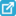View current table in a new window

### 3. Conclusion

There were changes of the maximum stress of the component with the three double-sided notches induced by the change of mutual distances of the individual mid notch. In case of the tensile loading process we have calculated shape factor α according the known formula for each change of the distance. The more increase of distance, the more reduction of shape factor α. It means that the value of loading force F will be reduced too. According the results we have found out that such theory works with the limited distance only. The maximum stress can be reduced till the notch does not exceed a certain minimum distance from the edge of the current sample. When it comes about the bending stress, the factor α was determined for the mutual distance 40 mm only. We have took into account that above constant for each calculation. We observed the change of maximum stress. According the modeled samples we have found out that values of maximum stress increased progressively. It follows that it is not possible to reduce the maximum stress value during the bending stress process with the constant force of mutual distance of notches. The main difference between the components with the one double-sided notch and the component with the tree double-sided notches is the maximum stress which depends on σc. The settings of SolidWorks problem or the factor α can cause that difference.

### Acknowledgements

This work was supported by the Ministry of Education of Slovakia Foundation under Grant VEGA No.1/0393/14, VEGA No.1/0731/16.

### References

  MURČINKOVÁ, Z.: Simulácia vrubových účinkov pomocou softvéru Pro/Mechanica. In: Trendy ve vzdělávání 2009 díl I: Technika, materiály, technologie a didaktika, Olomouc: VOTOBIA Olomouc, 2009. s. 149.In article  TREBUŇA, F. – ŠIMČÁK, F.: Odolnosť prvkov mechanických sústav. Košice: Emilena, 2004.In article  TREBUŇA, F. – JURICA, V. – ŠIMČÁK, F.: Pružnosť a pevnosť 2. Košice: Vienela, 2000.In article  www.ewp.rpi.edu/hartford/~ernesto/Su2012/EP/MaterialsforStudents/Aiello/Roark-Ch06.pdf.In article